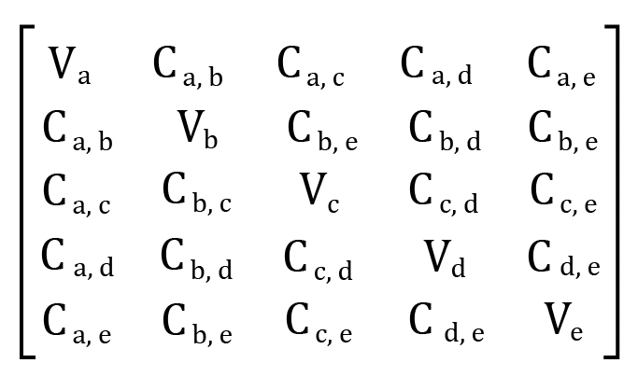Course Content

# Principal Component Analysis

1. What is Principal Component Analysis

3. Model Building

Principal Component Analysis

##Covariance Matrix

The next step is to create a covariance matrix. Why are we doing this? The covariance matrix allows us to see the relationship between variables in the dataset. If some variables have a strong correlation with each other, this will allow us to avoid redundant information in the next step. This is the meaning of the PCA algorithm: to make the differences between variables more pronounced, and to get rid of information overload.

The covariance matrix is a symmetric matrix of the form nxn, where n - is the total number of measurements, i.e. variables that we have in the dataset. If we have 5 variables: `x1`, `x2`, `x3`, `x4`, `x5`, then the covariance matrix 5x5 will look like this:Pay attention to the sign of the covariance values: if it is positive, then the variables are correlated with each other (when one increases or decreases, the second also), if it is negative, then the variables have an inverse correlation (when one increases, the second decreases and vice versa).

Let's use `numpy` to calculate the covariance matrix:

Read the dataset from the `train.csv` file (from web), standartize the data, calculate the covariance matrix, and display it.